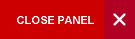# Department of Mathematics## Mathematics (Minor)

A minor in Mathematics requires passes in MATH1141, MATH1142, MATH1151, MATH1152 (or M10A/MATH1140 and M10B/MATH1150) at Level I, plus eighteen (18) advanced credits as indicated below:

Level II
MATH2402  Elements of Mathematics Analysis
MATH2410  A First Course in Linear Algebra

Level III
MATH3155  Complex Variables

Plus any two courses from the Math Elective List below:

MATHS ELECTIVE:
MATH2411  Introduction to ABstract Algebra
MATH2430  Linear Optimization
MATH2404  Intoduction to Probability Theory
STAT2001   Inferential Statistics
MATH2407 Stochastic Modelling
MATH2420  Ordinary Differential Equations
MATH2421 Fourier Series & Integral Transforms
MATH2403  Multivariable Calculus
MATH2431  Non-Linear Optimization
STAT3001  Regression Analysis
MATH3414  Selected Topics in Operations Research
MATH3402  A Course on Metric Space & Topology
MATH3421  Partial Differential Equations
MATH3422  Mathematical Modelling
MATH3434  Numerical Methods
MATH3401  Introduction to the Theory of Integration
MATH3403  Some Topics in Functional Analysis